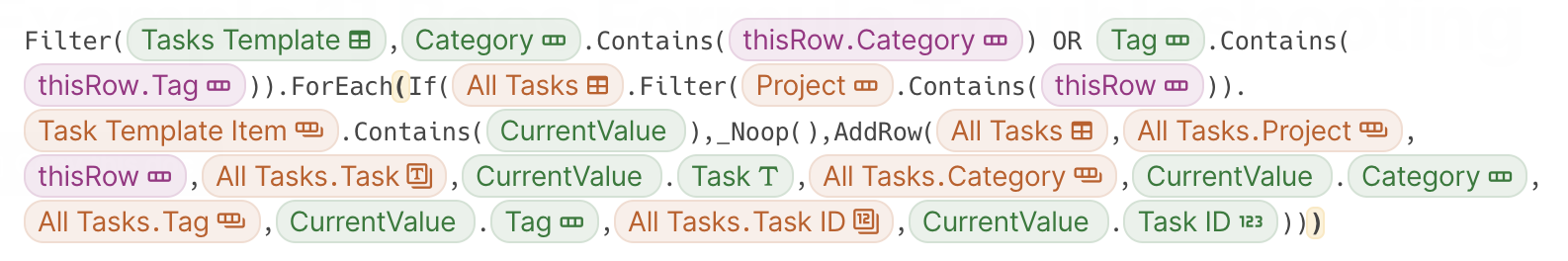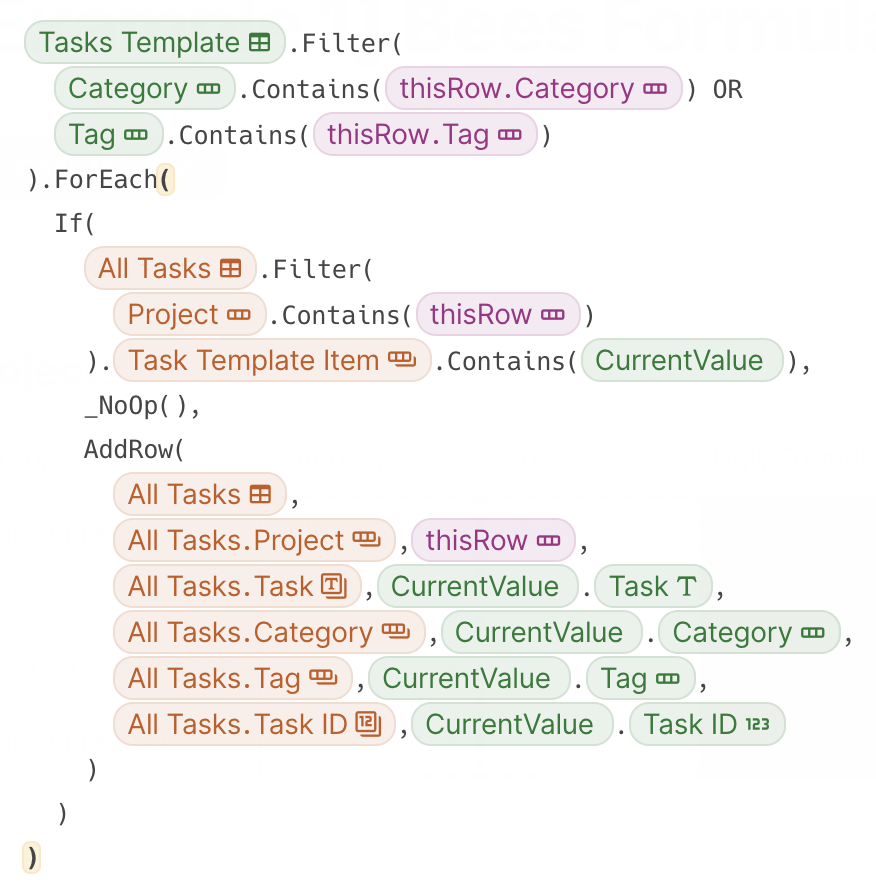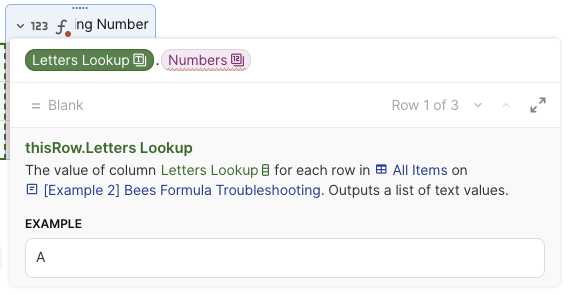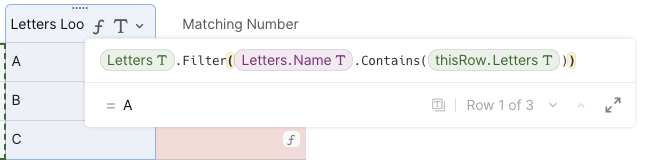Share
Explore#Good habits: how to write and troubleshoot big formulasGood habits: how to write and troubleshoot big formulas

A few patterns and a little structure can go a long way
I’ve spent the last several years working at Coda troubleshooting some of the biggest and most complex setups we’ve seen in customer docs. Here are some of the strategies I’ve picked up along the way for both writing and troubleshooting formulas.

# How to write a formula

I don’t know that I’ve ever seen one big formula. I have, however, seen groups of smaller formulas that look like one big formula. All big chunks can be broken down into smaller chunks and these smaller chunks are easier to understand.
When I’m overwhelmed, I take the time to rewrite the formula first. The following are patterns I’ve found to be helpful. Find what works best for you and keep in mind that each pattern should serve a purpose.## vs.## Use dot notation instead of parameters when possible

This makes it easier to see what table or list is being referenced and what the expression being assessed is. The more separation between the two, the easier it is to spot discrepancies.
When both the table to be filtered and the filter expression are inside the parentheses, it’s difficult to tell where one stops and the other starts.
`Filter([Table to filter], [Column 1] = [Some Value])`
Separating the two with dot notation makes it easier to separate and focus on either one individually.
`[Table to filter].Filter([Column 1] = [Some Value]) `

## Indent nested formulas

An If() statement for instance might contain formulas for each expression. Moving these to a new line and indenting them helps to highlight where the If() starts and where it finishes. New lines also help to separate where each expression in the If() statement starts and ends. This is true for any formula that can contain nested formulas, which is quite a few!
```If( [This expression is true], [Do this], [Else, do this] )```

## Match pairs

Some formulas have parameters that can be repeated in pairs. For these, I try to keep them on the same line to better spot what column I’m targeting and what value I’m placing in it, like an AddRow() button formula for example. Not only is it easier to read, it’s easier continue to build out the formula because the pattern stands out.
```AddRow( [Table to add a row to],```` [Table].[Column 1], [Value 1],`` [Table].[Column 2], [Value 2],`` [Table].[Column 3], [Value 3]``)`

## Bump closing parentheses to a new line

Dot notation allows us the ability to continue tacking on more functions. If we bump the closing parentheses of a formula to a new line, we can more clearly see the next formula.
`[Table of Data].Filter(`` [Column 1].Contains(thisRow.[Column 2])``).Count()`
In this example we can clearly see the objective is to count the items returned. If the intent is to find if that count is <, >, or = to some value, that will stand out clearly too.
`[Table of Data].Filter(`` [Column 1].Contains(thisRow.[Column 2])``).Count() > 0`

## Use spacing around comparison operators(<, >, or =)

Adding a little room around comparison operators helps to separate the values and formulas you’re assessing. When everything is crunched together, it’s easy for your eye to skim right past a single character operator. Adding space helps these stand out, and the larger the formula you’re troubleshooting, the more this helps.

## AND & OR logical operators use new lines

Logical operators sit in between expressions. Using new lines for each expression can help you focus on each one individually, as well as the relationship between them.
`[Table of Data].Filter(`` [Column 1].Contains(thisRow.[Column 2]) AND `` [Column 2] > [Value] AND `` [Column 3] = User()``)`

# Troubleshooting

## Divide and conquer

As I mentioned in the first line of this doc, big formulas are just aggregations of smaller formulas. So take advantage of that and split them up when certain parts get overwhelming.

### Use worker columnswork·er col·umn /ˈwərkər ˈkäləm/
A column added to a Coda table that calculates a smaller formula needed within a larger formula used elsewhere
Something Ben adds to a table when he wants to make formulas look and run better
There’s no need to overload a single formula. Using worker columns can help divide up the complexity by breaking things down into digestible chunks, and it can make a doc more efficient.
Formulas are part of a dependency graph, and if a value changes that the formula references, it recalculates. The more values referenced in a single formula, the more likely it is to have to recalculate when any change is made in the doc. Splitting up larger formulas into smaller formula columns can break up these dependencies and allow more values to be cached.

### Test table filters and disable if formulas in a column or canvas formula

Both table filter formulas and disable if formulas need to evaluate to true or false. It’s difficult to see if that’s what’s happening while in the button builder, for instance. Pulling these out into a column will show the results for each row. If the results are returning values instead of true or false, that’s one check. The next check is whether or not the rows are returning the expected true or false result. Not only does this make spotting an issue easier, it makes testing and fixing the issue easier too.

### Check a broken formula one piece at a time

If a big formula isn’t returning the right value, check each part individually to single out the expression that needs a little more work.
If you’re starting with this
`[Table of Data].Filter(`` [Column 1].Contains(thisRow.[Column 2]) AND `` [Column 2] > [Value] AND `` [Column 3] = User()``)`
Try checking this first
`[Table of Data].Filter(`` [Column 1].Contains(thisRow.[Column 2])``)`
Then move to this
`[Table of Data].Filter(`` [Column 2] > [Value]``)`
And finally check this
`[Table of Data].Filter(`` [Column 3] = User()``)`
Chances are, you’ll be able to single out the issue and more easily test the fix as well.

Formulas can reference other columns that also have formulas. Sometimes the cause of the issue isn’t in the formula you’re assessing.

### Check the chipsIn the above screenshot, the error line is under Numbers, but this is a simple formula and this seems to signify a disconnect of some sort. We can click on the Letters Lookup and see where that column is, then visit it and see if there’s an issue.

## Formula builder hints

Now that we followed the breadcrumbs, we need to focus in on the hints to find the issue.### Where the hints live

Chips vs. text - Chips represent columns, tables, canvas controls, and canvas formulas in Coda’s formula language. This makes it easy to see at a glance if you’re referencing a variable or if you should be seeing text.
Chip colors - Coda color codes chips to show where values are coming from. The same color means those variables are from the same table.
Chip icons - Icons show not only the data type, but also whether the data being returned for that chip is singular or multiple. The icon shows the data type and the icon stacked means it can return multiple values.
Highlighted parentheses - When you place your cursor next to a parentheses in the formula builder, it and it’s closing or opening pair will highlight in yellow.
Cursor placement to prompt help text - Placing your cursor in or next to a formula in the formula builder will prompt help info to pop up just under the builder. As you arrow through the formula, parameters will highlight to let you know what it’s expecting to see.
For the example above, the chip icons give us the hint we need to find the issue. If you look at the first Letters chip, the icon is a single text T, but a filter takes a list of items. The original formula we’re solving for is using dot notation as if to extract a column value from a row value. So instead of feeding the filter a single text value, the intent was probably to feed it a table of rows.
This happened from a table and a column having the same name and choosing the wrong one in the formula builder.

# Wrap up

At some point you’re likely to need to revisit a formula you wrote long ago and will have to think back through your process, and at that point, having taken a little time up front to give it a nice layout flow can really pay off.

### Thank you for reading and I’m excited to see what you all build next!